# Database Constraints

 Now let me show how the ideas I've been discussing can be used in the formulation of database constraints, using examples from Chapter 6. Please note that the formulations shown aren't the only ones possible, in general. Example 1 Status values must be in the range 1 to 100 inclusive. ` CONSTRAINT C1 FORALL s ( IF s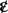S THEN s.STATUS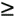0 AND100 ) ; ` ("For all possible values s, if s is a tuple in relvar S, then the STATUS value within that tuple must be in the specified range"; note the dot-qualified attribute references, and recall from Chapter 5 that "s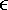S can be read as "s [is] in S"). Now, I'm sure you can see that many constraints are going to involve a proposition of the general form just illustrated: ` FORALL t ( IF tp ) ` (where p is some predicate). We can simplify such propositions slightly by defining the following as a shorthand: ` FORALL tp ) ` The bound variable t here is said to range over (the current value of) relvar R, and the expression FORALL trange-coupled quantifier. Using this shorthand, constraint C1 becomes: ` CONSTRAINT C1 FORALL sS ( 0 AND100 ) ; ` In the same kind of way, it's helpful to define the expression: ` EXISTS tp ) ` to be shorthand for the following: ` EXISTS t ( ( tp ) ) ` In fact, we can (and I will) simplify matters still further, by allowing the ranges for bound variables to be defined external to the expressions that use those bound variables. For example: ` s RANGES OVER { S } ` Now I can reduce constraint C1 to just:[*][*] SQL allows a simple constraint like this one to be reduced still further by (in effect) eliding the quantifier. To be precise, we can specify a "CHECK constraint" as part of the definition of base table S that looks like this: CONSTRAINT C1 CHECK (STATUS >= 0 AND STATUS <= 100). We can even elide that "CONSTRAINT C1" as well, if we want. ` CONSTRAINT C1 FORALL s ( s.STATUS0 AND100 ) ; ` For the remainder of this section (and the next), I'll assume the following range definitions are in effect: ` s RANGES OVER { S } x RANGES OVER { S } y RANGES OVER { S } p RANGES OVER { P } sp RANGES OVER { SP } ` I'll also introduce additional bound variables as and when necessary. Example 2 Suppliers in London must have status 20. ` CONSTRAINT C2 FORALL s ( IF s.CITY = 'London' THEN s.STATUS = 20 ) ; ` Example 3 Supplier numbers are unique. ` CONSTRAINT C3 FORALL x, y ( IF x.SNO = y.SNO THEN x.SNAME = y.SNAME AND x.STATUS = y.STATUS AND x.CITY = y.CITY ) ; ` The quantifier FORALL x,y is shorthand for FORALL x FORALL y. Example 4 No supplier with status less than 20 can supply part P6. ` CONSTRAINT C4 FORALL s ( IF s.STATUS < 20 THEN FORALL sp ( IF sp.SNO = s.SNO THEN sp.PNO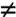PNO('P6) ) ) ; ` Example 5 Every shipment must have a supplier. ` CONSTRAINT C5 FORALL sp ( EXISTS s ( sp.SNO = s.SNO ) ) ; ` Example 6 No supplier number appears in both relvar LS and relvar NLS. ` CONSTRAINT C6 FORALL mLS FORALL NLS ( Example 7 Supplier S1 and part P1 must never be in different cities. CONSTRAINT C7 NOT ( EXISTS s EXISTS p ( s.SNO = SNO('S1') AND p.PNO = PNO('P1') AND s.CITYI'll show a few more examples (not based on examples in Chapter 6) in order to illustrate some additional points. Example 8 There must always be at least one supplier. CONSTRAINT C8 EXISTS s ( TRUE ) ; Example 9 All parts are either blue or green, and there exists at least one part. CONSTRAINT C9 FORALL p ( p.COLOR = 'Blue' OR p.COLOR = 'Green' ) AND EXISTS p ( TRUE ) ; Note the use of two distinct bound variables here, both called p. Example 10 All parts are either blue or green, or there exists at least one red part. CONSTRAINT C10 FORALL p ( p.COLOR = 'Blue' OR p.COLOR = 'Green' ) OR EXISTS p ( p.COLOR = 'Red' ) ; `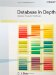Database in Depth: Relational Theory for Practitioners
ISBN: 0596100124
EAN: 2147483647
Year: 2006
Pages: 127
Authors: C.J. Date

Similar book on Amazon